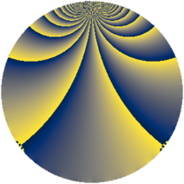# Properties

 Label 280.2.bfLevel $280$ Weight $2$ Character orbit 280.bf Rep. character $\chi_{280}(109,\cdot)$ Character field $\Q(\zeta_{6})$ Dimension $88$ Newform subspaces $1$ Sturm bound $96$ Trace bound $0$

# Learn more

## Defining parameters

 Level: $$N$$ $$=$$ $$280 = 2^{3} \cdot 5 \cdot 7$$ Weight: $$k$$ $$=$$ $$2$$ Character orbit: $$[\chi]$$ $$=$$ 280.bf (of order $$6$$ and degree $$2$$) Character conductor: $$\operatorname{cond}(\chi)$$ $$=$$ $$280$$ Character field: $$\Q(\zeta_{6})$$ Newform subspaces: $$1$$ Sturm bound: $$96$$ Trace bound: $$0$$

## Dimensions

The following table gives the dimensions of various subspaces of $$M_{2}(280, [\chi])$$.

Total New Old
Modular forms 104 104 0
Cusp forms 88 88 0
Eisenstein series 16 16 0

## Trace form

 $$88q - 2q^{4} - 40q^{9} + O(q^{10})$$ $$88q - 2q^{4} - 40q^{9} + 6q^{10} - 6q^{14} + 4q^{15} + 2q^{16} - 24q^{20} - 8q^{24} - 2q^{25} - 14q^{26} + 14q^{30} - 28q^{31} + 24q^{34} + 60q^{36} - 16q^{39} - 32q^{40} - 16q^{41} - 2q^{44} - 42q^{46} - 8q^{49} - 12q^{50} + 8q^{54} - 28q^{55} + 36q^{56} + 10q^{60} + 52q^{64} - 12q^{65} - 44q^{66} - 48q^{70} + 16q^{71} - 42q^{74} - 36q^{76} - 4q^{79} - 40q^{80} - 20q^{81} + 12q^{84} - 24q^{86} + 4q^{89} - 96q^{90} - 22q^{94} - 34q^{95} + 80q^{96} + O(q^{100})$$

## Decomposition of $$S_{2}^{\mathrm{new}}(280, [\chi])$$ into newform subspaces

Label Dim. $$A$$ Field CM Traces $q$-expansion
$$a_2$$ $$a_3$$ $$a_5$$ $$a_7$$
280.2.bf.a $$88$$ $$2.236$$ None $$0$$ $$0$$ $$0$$ $$0$$